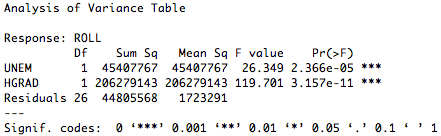Want to share your content on R-bloggers? click here if you have a blog, or here if you don't.

The commonly applied analysis of variance procedure, or ANOVA, is a breeze to conduct in R. This tutorial will explore how R can be used to perform ANOVA to analyze a single regression model and to compare multiple models.

### Tutorial Files

Before we begin, you may want to download the sample data (.csv) used in this tutorial. Be sure to right-click and save the file to your R working directory. This dataset contains information used to estimate undergraduate enrollment at the University of New Mexico (Office of Institutional Research, 1990). Note that all code samples in this tutorial assume that these data have already been read into an R variable and have been attached.

### Pre-ANOVA Steps

Prior to running ANOVA, we need to have one or more regression models. In the segments on simple linear regression and multiple linear regression, we created a series of models using one, two, and three predictors to estimate the fall undergraduate enrollment at the University of New Mexico. The complete code used to derive these models is provided in their respective tutorials. This article assumes that you are familiar with these models and how they were created. Therefore, a shorthand method for generating the models is displayed below.

1. > #create three linear models using lm(FORMULA, DATAVAR)
2. > #one predictor model
3. > onePredictorModel <- lm(ROLL ~ UNEM, datavar)
4. > #two predictor model
5. > twoPredictorModel <- lm(ROLL ~ UNEM + HGRAD, datavar)
6. > #three predictor model
7. > threePredictorModel <- lm(ROLL ~ UNEM + HGRAD + INC, datavar)

### One Model ANOVA

In R, the anova(MODEL) function can be used to run ANOVA, where MODEL is the variable containing the model to be analyzed. The output of the anova(MODEL) function is a standard ANOVA table. An example of how to use the anova(MODEL) function is demonstrated below.

1. > #use anova(MODEL) to create an ANOVA table for a given model
2. > #what are the ANOVA results for the two predictor model?
3. > anova(twoPredictorModel)

The output of the preceding function is pictured below. A similar procedure could be followed to produce ANOVA tables for the one and three predictor models.### Multiple Model ANOVA Comparison

ANOVA can also be used to compare successive models. The following code demonstrates how to do this using the anova(MODEL1, MODEL2, … MODELi) function, where MODEL1, MODEL2, etc. are all model variables.

1. > #use anova(MODEL1, MODEL2, … MODELi) to compare successive models
2. > #how do the one predictor, two predictor, and three predictor models compare to one another according to ANOVA?
3. > anova(onePredictorModel, twoPredictorModel, threePredictorModel)

The output of the preceding function is pictured below. These results give us one context in which to compare the models.### Complete ANOVA Table Example

To see a complete example of how ANOVA tables can be generated in R, please download the ANOVA tables example (.txt) file.

### References

Office of Institutional Research (1990). Enrollment Forecast [Data File]. Retrieved November 22, 2009 from http://lib.stat.cmu.edu/DASL/Datafiles/enrolldat.html